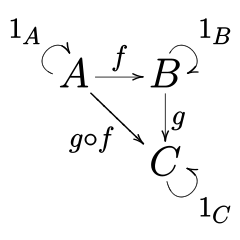# Category Theory Notes

By Xah Lee. Date: .

Category theory formalizes mathematical structure and its concepts in terms of a labeled directed graph called a category, whose nodes are called objects, and whose labelled directed edges are called arrows (or morphisms).

A category has two basic properties: the ability to compose the arrows associatively and the existence of an identity arrow for each object.

## narrow definition of category

A category is:

• a collection of sets.
• a collection of bijection functions, that maps a set to another.

such that the following is true:

• (associativity) if f : a → b, g : b → c and h : c → d then h ∘ (g ∘ f) = (h ∘ g) ∘ f
• (identity) for every set, there is a identity function that maps the set to itself.category diagram. A B C, each is a set. f and g are functions, represented by arrows. g ∘ f is function composition. It maps A to C because the associativity requirement. Looped arrows are identity functions, also required.

more generally, the sets are called “objects”, and the objects are usually some structure, such as groups.

and the function are called morphism (or arrows), and the function are often those that preserve some structure of the object. e.g. if the objects are groups, then the functions are homomorphisms.

Another example: if the objects are topological spaces, then the function are continuous functions.

so, for each class of objects, we use a particular class of functions. So, we have different categories, and usually we give each a name.

here's a table

category nameobjectmorphisms
Magmagmasmagma homomorphisms
Manpsmooth manifoldsp-times continuously differentiable maps
Metmetric spacesshort maps
R-ModR-modules, where R is a ringR-module homomorphisms
Ringringsring homomorphisms
Setsetsfunctions
Grpgroupsgroup homomorphisms
Toptopological spacescontinuous functions
Uniuniform spacesuniformly continuous functions
VectKvector spaces over the field KK-linear maps

[ Category theory ] [ 2018-03-28 https://en.wikipedia.org/wiki/Category_theory ]

a textbook: https://arxiv.org/abs/1803.05316